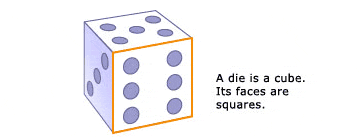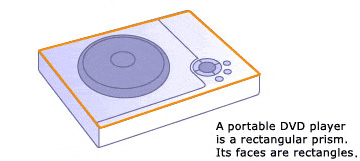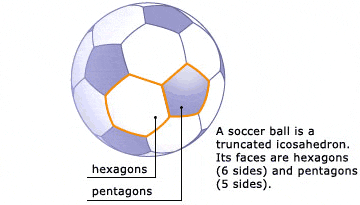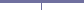# 3D Shapes

There are many types of three-dimensional shapes. You've surely seen spheres and cubes before. In this lesson, you'll learn about polyhedra — three-dimensional shapes whose faces are polygons — and you'll also learn about two special types of polyhedra: prisms and pyramids.

Polyhedra

A die is in the shape of a cube. A portable DVD player is in the shape of a rectangular prism. A soccer ball is in the shape of a truncated icosahedron. These shapes are all examples of polyhedra.

A three-dimensional shape whose faces are polygons is known as a polyhedron. This term comes from the Greek words poly, which means "many," and hedron, which means "face." So, quite literally, a polyhedron is a three-dimensional object with many faces.

The faces of a cube are squares. The faces of a rectangular prism are rectangles. And the faces of a truncated icosahedron are pentagons and hexagons — there are some of each.

The other parts of a polyhedron are its edges, the line segments along which two faces intersect, and its vertices, the points at which three or more faces meet.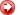Next: